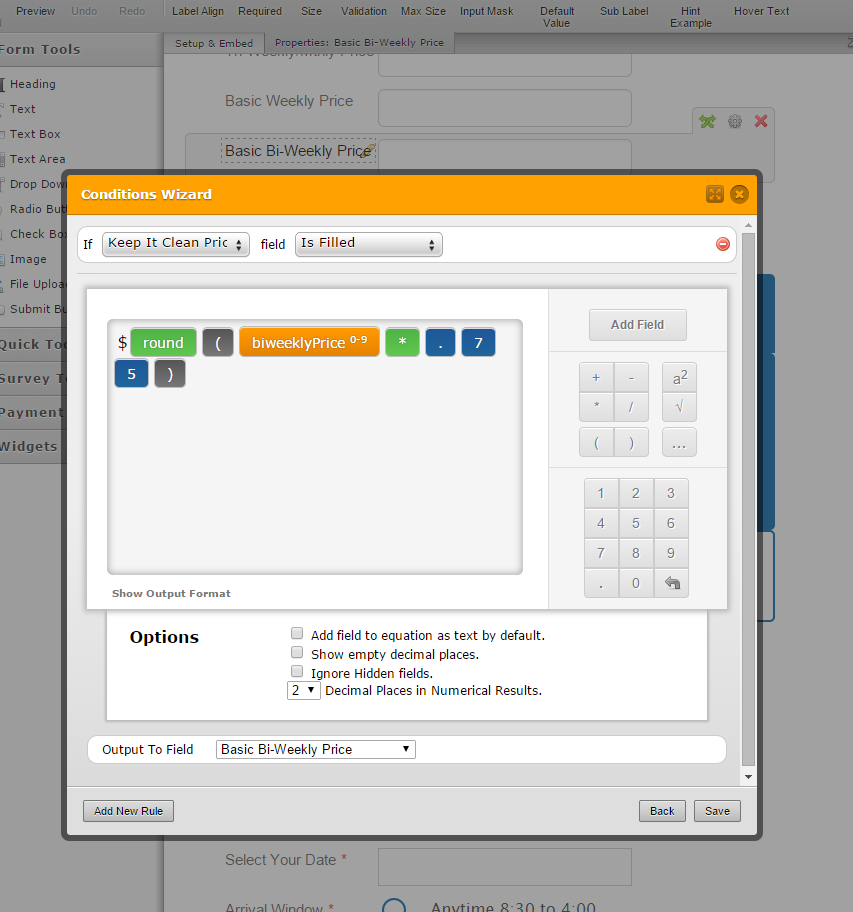# How do I set minimum value to the calculated result?

•kauseway
Asked on March 19, 2015 at 09:36 AM

I have a form that calculates pricing for cleans based on home size.  But the calculation sometimes is \$60.  Our minimum cost is \$75. Is there a way for it to default to minimum amount if the calculation is not minimum?

http://form.jotformpro.com/form/50005155818954

Page URL:
http://form.jotformpro.com/form/50005155818954•Kiran
Answered on March 19, 2015 at 11:54 AM

You may add a condition to the calculation field to check if the final price is less than \$75 to replace with \$75.I see that you have used textbox field for calculating using the conditions. The less than and greater than conditions cannot be set using textbox fields. This textbox field needs to be replaced with a calculator widget or a number field so that you'll be able to set a condition using less than or greater than.

Hope this information helps. Please let us know if you need any further assistance. We will be happy to help.

Thanks!

•ecofrogclean
Answered on September 19, 2015 at 07:24 PM

Hi there,

I am trying to do this exact thing. Where I get stuck is that if the calculated value is above the "less than" rule that I've set, it doesn't go above the minimum value condition.

So if the condition I have set is if the calculation is less than 2.5, to set at 2.5. However if it's greater than 2.5, it's still set at 2.5.

http://www.jotform.ca/form/52538551027252

•BDAVID
Answered on September 20, 2015 at 04:54 PM

Remove the dollar sign "\$" from the calculation, and add a third calculation field, like this:Create conditions like this:Here is my clone version: http://form.jotform.co/form/52626583924867

Let us know if you need more help.